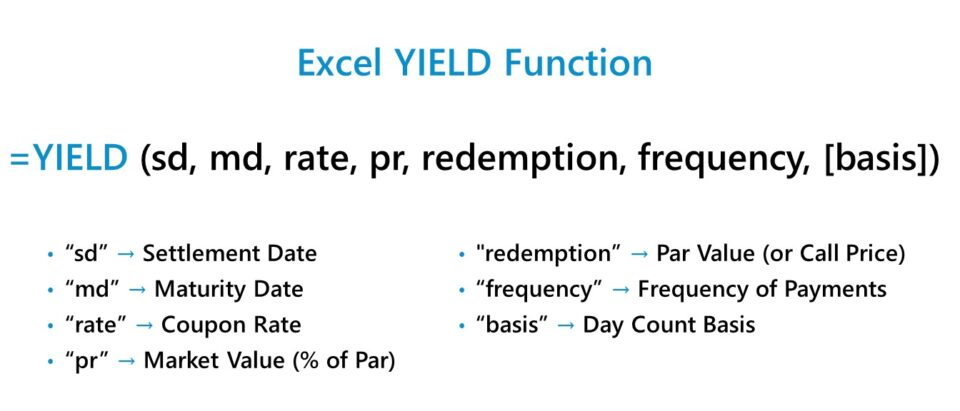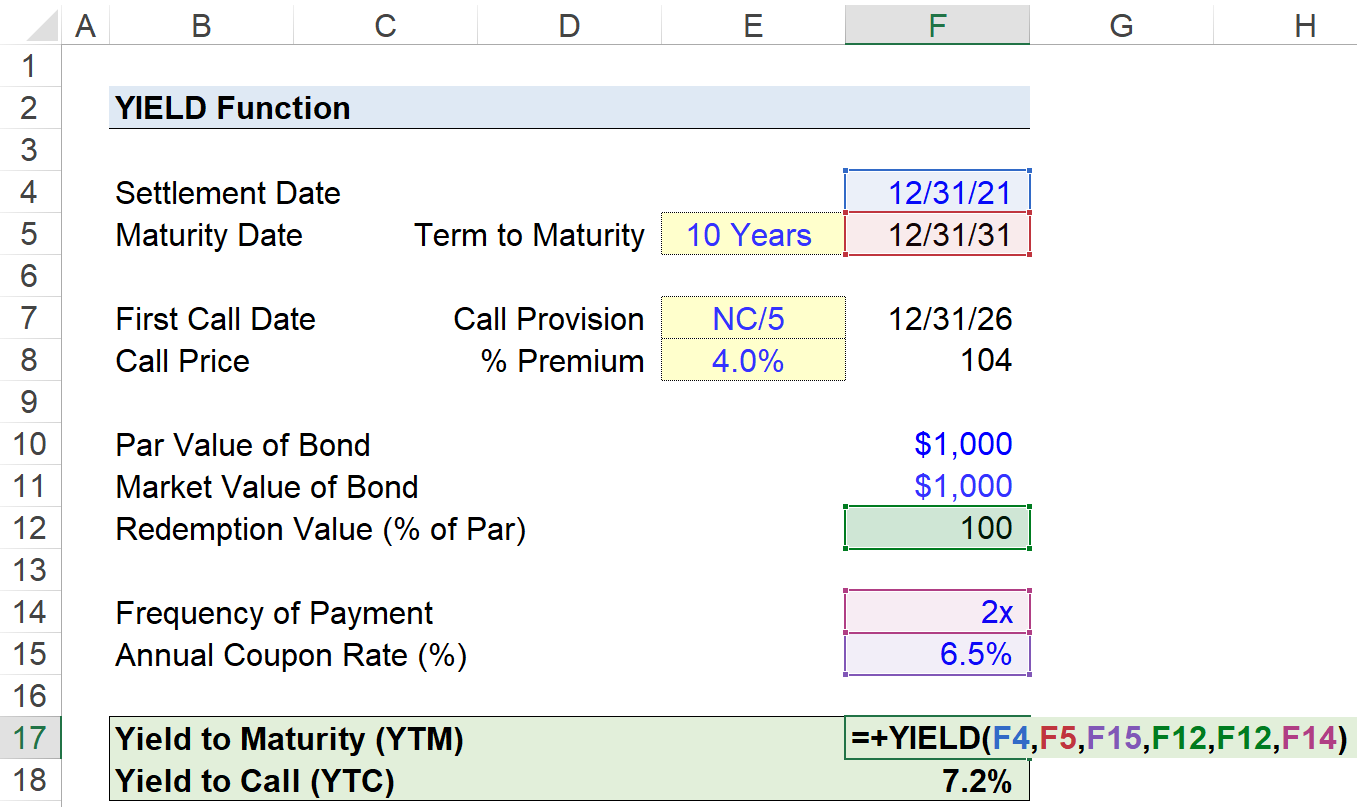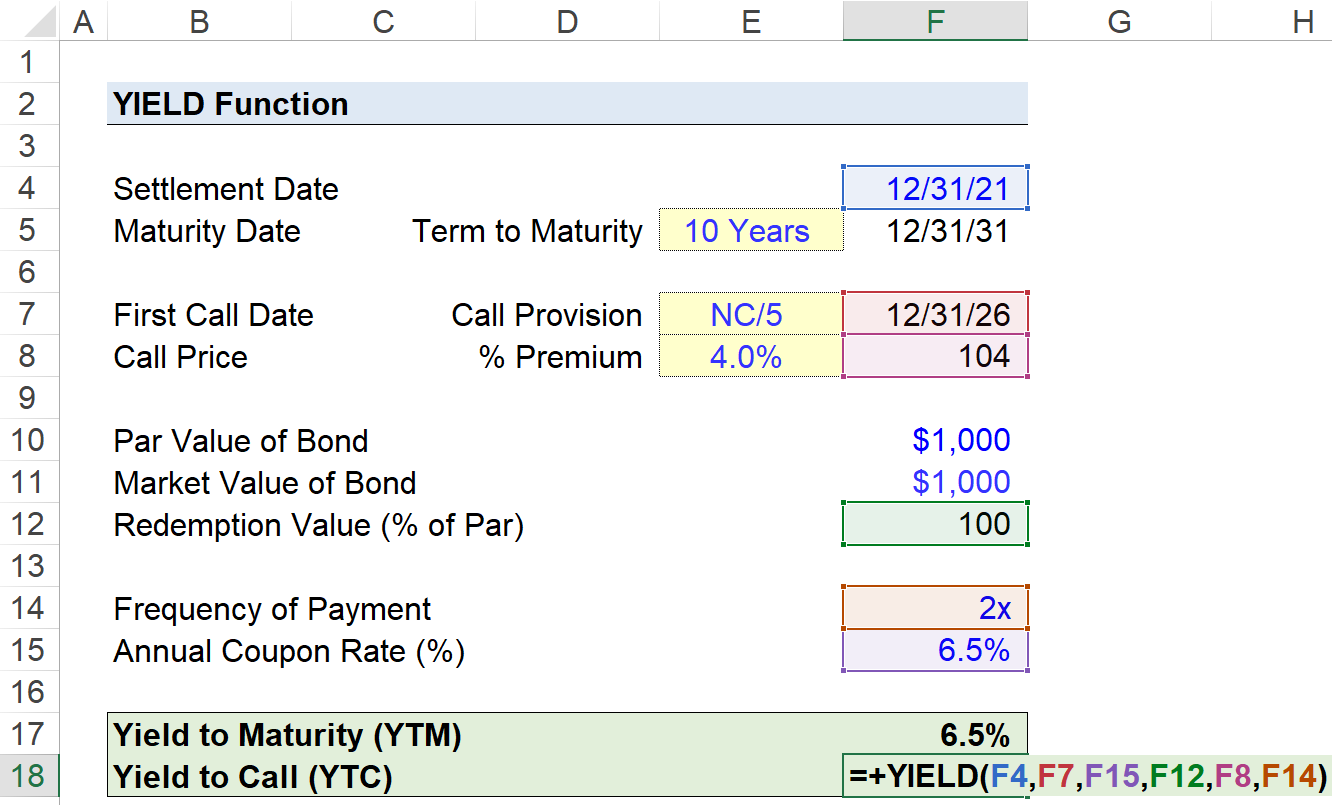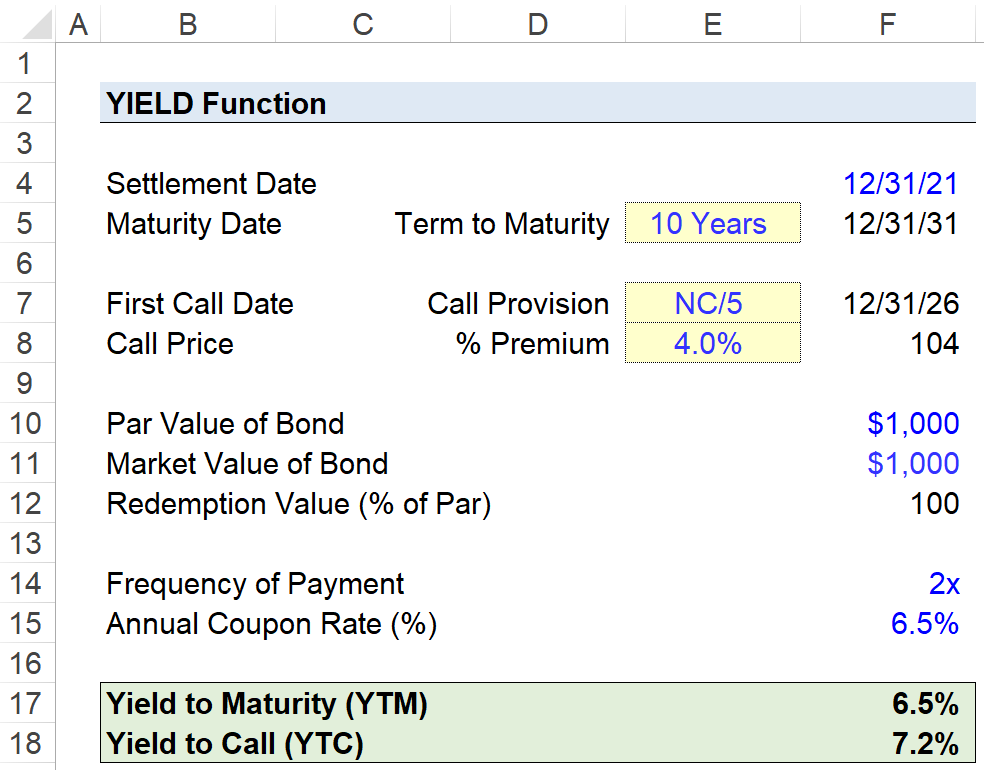Welcome to Wall Street Prep! Use code at checkout for 15% off.# YIELD Function

Guide to Understanding the "YIELD" Function in Excel## How to Use YIELD Function (Step-by-Step)

The Excel “YIELD” function is most often used in practice to determine the yield on a bond or a related debt instrument.

As part of a financing arrangement, the borrower is obligated to pay periodic interest payments to the lender until the date of maturity, in which the remaining balance of the principal must be repaid in full.

Before the terms of the lending agreement are formalized, i.e. prior to the settlement date, the yield earned on a bond by the lender can be estimated. However, there is substantial room for variance between the expected and the actual return.

Therefore, lenders must rely on multiple bond yield metrics, rather than merely one, to measure their potential returns under different scenarios.

## Bond Terminology Review

Before delving further into the Excel “YIELD” function, it is necessary to preface the section with a review of common bond terminology to not only understand the calculation itself, but also to reduce the chance for misinterpretations.

Term Definition
Par Value (or Face Value)
• The par value of a bond, often referred to as the face value (FV), is the stated price on the date of original issuance.
Market Value
• The market value refers to the price of a security on the present date, i.e. the pricing as set by the supply and demand in the market.
Maturity
• The term maturity, or “tenor”, is the length of the borrowing term agreed upon by the lender and borrower, in which the obligations on both sides are expected to continue, such as the coupon payments on a bond.
Par Bond vs. Discount Bond vs. Premium Bond
1. Par Bond: If the par value of a bond is \$1,000 (“100”) and the market price of the bond is also \$1,000, then the bond is trading at par.
2. Discount Bond: If the par value of a bond is \$1,000 (”100”) and the market price is currently \$900 (“90”), then the security is trading at a discount, i.e. trading below par.
3. Premium Bond: If the par value of a bond is \$1,000 (“100”) yet the market price is \$1,100 (“110”), then the bond is selling at a premium and trading above par.
Coupon Rate (or Interest Rate)
• The coupon rate is the interest rate attached to a bond, i.e. the cost of the borrowing.
• The coupon rate is multiplied by a bond’s par value to calculate the annual coupon payment owed to the issuer of the bond.
Current Yield
• The current yield is the annual return of a bond, assuming that the security is held for the next year.
Yield to Maturity (YTM)
• The yield to maturity (YTM) represents the annual rate of return anticipated to be earned on a bond, assuming that the security is held until the date of maturity.
Yield to Call (YTC)
• The yield to call (YTC) is the return on a callable bond, assuming the bondholder decides to redeem the bond on the earliest call date, i.e. repayment prior to maturity.
Yield to Worst (YTW)
• The yield to worst (YTW) is the minimum return earned on a callable bond, i.e. the floor yield, aside from the yield if the issuer were to default.
Callable Bond vs. Non-Callable Bond
• If a bond issuance is callable, the lending agreement will contain a provision that the issuer can redeem the borrowing before maturity; however, the option to pay off the debt earlier comes at a cost.
• Most callable bonds have an initial set number of years in which early repayment of the bond is not permitted, as well as fees for paying the bond back earlier than originally agreed upon.
• All else being equal, a callable bond should exhibit a higher yield than a comparable non-callable bond.

## Excel YIELD Function Formula

The formula for using the YIELD function in Excel is as follows.

= YIELD (settlement, maturity, rate, pr, redemption, frequency, [basis])

Where:

• settlement” = Settlement Date
• maturity” = Original Maturity Date or Early Redemption Date
• rate” = Annual Coupon Rate (%)
• pr” = Bond Quote (% of Par)
• redemption” = Par Value or Call Price
• frequency” = Number of Compounding Periods (e.g. Annual = 1x, Semi-Annual = 2x, Quarterly = 4x)
• basis” = Day Count Basis

The brackets around the final input, “basis”, denotes that it can be omitted, i.e. left blank.

In order for the implied yield to be accurate, the units of time used in the equation must remain consistent all throughout the calculation.

For instance, if a borrower has taken out a ten-year bond with an annual interest rate of 10% paid on a semi-annual basis, the time-adjusted (i.e. semi-annual) interest rate on the bond is 5.0%.

• Semi-Annual Interest Rate (rate) = 10% ÷ 2 = 5.0%

## YIELD Function Excel Syntax

The table below describes the syntax of the Excel YIELD function in more detail.

Argument Description Required?
settlement
• The settlement date of the security, i.e. the date on which the bond financing arrangement begins.
• Required
maturity
• The maturity date of the security is the date on which the agreement between the borrower and lender is expected to end.
• No more coupon payments remain, and the original principal was repaid in full, assuming the borrower did not default.
• Required
rate
• The annual coupon rate, i.e. interest rate, which determines the amount owed to the lender per annum.
• Required
pr
• The quoted price of the security per the par value (”100”).
• Required
redemption
• The redemption value, i.e. assuming either prepayment or payment at the originally stated maturity, of the security per the par value (”100”).
• Required
frequency
• The number of coupon payments issued per year. e.g. annual, semi-annual, or quarterly.
• Required
basis
• The day count basis as stated in the lending agreement.
• “0” = 30 / 360 (”Omitted”)
• “1” = Actual / Actual
• “2” = Actual / 360
• “3” = Actual / 365
• “4” = European 30 / 360

(Source: Microsoft)

• Optional

## YIELD Function Calculator – Excel Model Template

We’ll now move on to a modeling exercise, which you can access by filling out the form below.Submitting...

## Step 1. Bond Yield Calculation Exercise Assumptions

Suppose we’re tasked with calculating the yield on a ten-year semi-annual corporate bond given the following set of assumptions:

• Settlement Date = 12/31/21
• Term to Maturity = 10 Years
• Maturity Date = 12/31/31

The structure of the corporate bond issuance has a stated maturity of ten years and is not callable for the first five years of the borrowing term (i.e. NC/5).

## Step 2. Call Provision Assumptions

The first call date is therefore five years from the date of original issuance, with a call premium of 4.0%.

• Call Provision = NC/5
• First Call Date = 12/31/26
• Call Premium (%) = 4%
• Call Price = 104

The bond was issued at par, i.e. “100”, and is trading in the markets at the same price as of the present date.

## Step 3. Frequency of Payment and Coupon Rate Assumptions

The final two assumptions left are the frequency of payment and the coupon rate (%).

Earlier we stated the issuance was a semi-annual bond, so the frequency of payment is 2 times per annum.

The annual coupon rate that we’ll assume is 6.5%, meaning that the annual coupon is \$65

• Frequency of Payment = 2x
Annual Interest Rate = 6.5%

The primary factors that determine the yield on bonds are the par value, coupon rate (%), the coupon (\$), and the borrowing term.

Furthermore, the price of a bond and the yield are inversely related, wherein if the price of a bond were to rise, its yield would decline (and vice versa).

## Step 4. Bond Yield Calculation in Excel

With our assumptions complete, we can now calculate the yield to maturity (YTM) and yield to call (YTC) of the bond.

The inputs entered in our YIELD function formula to compute the yield to maturity (YTM) are as follows.

=YIELD(F4,F7,F15,F12,F8,F14)The implied yield to maturity (YTM), i.e. the return if held until the date of maturity, is 6.5% on the bond.

In contrast, the formula to calculate the yield to call (YTC) is the following.

=YIELD(F4,F7,F15,F12,F8,F14)The distinction of YTC from the prior YTM calculation, which has been formatted in italics, is the maturity date and the call price:

1. Maturity Date → The bondholder is assumed to have called the bond earlier than anticipated on the first call date and paid a premium.
2. Call Price → The call price becomes relevant only for callable bonds, in which a call premium (i.e. fee) is attached to compensate the lender for the loss of the future interest payments and the risk of needing to reinvest the capital.

Using the YIELD function in Excel, we arrived at an implied yield to maturity (YTM) is 6.5%, whereas the yield to call (YTC) was 7.2% in comparison.

The bondholder in our hypothetical scenario—assuming that earning a higher return is the priority—is likely to call the bond early because of the higher yield.Turbo-charge your time in Excel Used at top investment banks, Wall Street Prep's Excel Crash Course will turn you into an advanced Power User and set you apart from your peers.
Inline Feedbacks